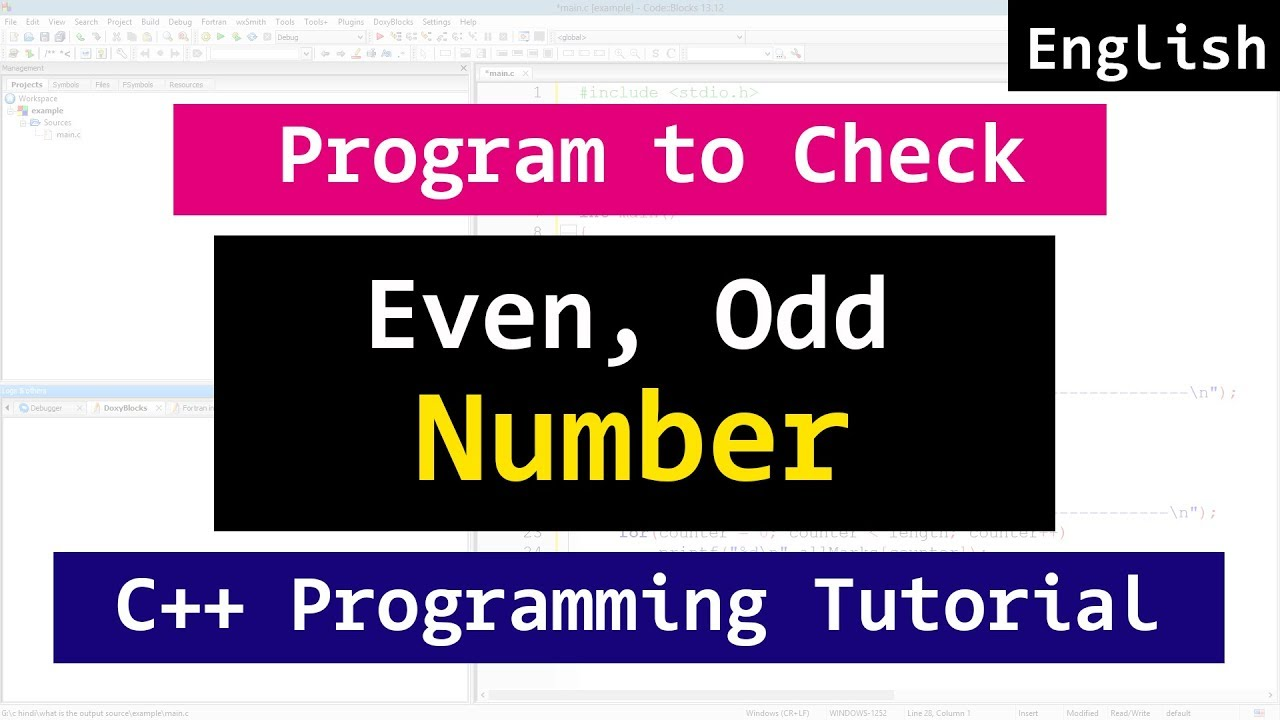# C++ Even or Odd Number Program | CPP video TutorialIn this c++ video tutorial you will learn to write a cpp program to check whether the number entered by the user is even or odd in detail with example.

An even number is an integer which is evenly divisible by 2. if the number is not even number then it is an odd number.

Here in this program we are using the modulus ( % ) operator to get the remainder and then we are using if statements to printout the results.

Watch this tutorial in Hindi at

Subscribe for more tutorials